# Numbers - math word problems

#### Number of problems found: 1462

• Barrel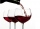Barrel of wine is filled by 9/14. What part of the barrel will remain filled when 1/4 of the wine we pour from the barrel?
• School trip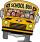School trip cost 247.2 Eur for one class (24 students). How much would cost a trip for two classes? (both classes together have 53 students)
• Garden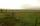The garden has two opposite parallel fences. Their distance is 33.1 m. Lengths in these two fences are 75.5 meters and 49.4 meters. Calculate the area of this garden.
• Three members GPThe sum of three numbers in GP (geometric progression) is 21 and the sum of their squares is 189. Find the numbers.
• Small painters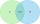There are ten pupils in the painting group. Eight pupils paint with watercolors and nine pupils with tempera colors. How many pupils paint both water and tempera colors when each pupil paints?
• DivisorsThe sum of all divisors unknown odd number is 2112. Determine sum of all divisors of number which is twice of unknown numbers.
• Dance groupThe dance group formed groups of 4, 5, and 6 members. Always one dancer remains. How many dancers were there in the whole group?
• Television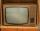When we add to the difference between the numbers 1704 and 654 the difference numbers 14920 and 14042, we get a year in which television was invented. How many years people known the TV?
• Exhibition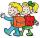The teacher paid for 280 Kč for 4.A students for admission to the exhibition. How many students were on the exhibition?
• Napkins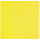Viera and Darina exchanged napkins. Always 3 yellow for 4 red napkins. How many yellow napkins will Viera receive for 24 red napkins?
• The fastestThe fastest boat can reach speeds more than 710% as fast as the Queen Mary 2. How would you express this number as a fraction and as a decimal?
• Operations with sets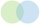The set B - A has twice as fewer elements than the set A - B and four times fewer elements than the set A ∩ B. How many times more elements does the set A have than the set B?
• Star equationWrite digits instead of stars so that the sum of the written digits is odd and is true equality: 42 · ∗8 = 2 ∗∗∗
• QuotientFind quotient before the bracket - the largest divisor 51 a + 34 b + 68 121y-99z-33
• RectangleIn rectangle ABCD with sides |AB|=19, |AD|=16 is from point A guided perpendicular to the diagonal BD, which intersects at point P. Determine the ratio ?.
• Fruits 2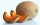An apple costs 7p and a melon costs seven times more. How much will 6 apples and 2 melons cost
• Jansen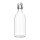Jansen had 1.3 litres of fruit juice. He drank 0.5 litres during lunch. How many litres of fruit juice does he have left?
• BonusThe gross wage was 1323 USD including 25% bonus. How many USD were bonuses?
• Florist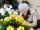Florist has 84 red and 48 white roses. How many same bouquets can he make from them if he must use all roses?
• Landlord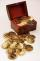Landlord had 49 ducats more than Jurošík. How many ducats Jurošík steal landlord if the Jurošík now 5 ducats more?

Do you have an interesting mathematical word problem that you can't solve it? Submit a math problem, and we can try to solve it.

We will send a solution to your e-mail address. Solved examples are also published here. Please enter the e-mail correctly and check whether you don't have a full mailbox.

Please do not submit problems from current active competitions such as Mathematical Olympiad, correspondence seminars etc...